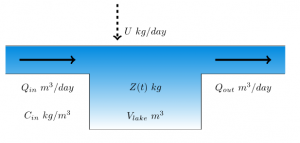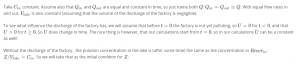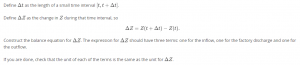# 1.7 Mathematical model

Course subject(s) 1. Introducing Mathematical Modelling

In the last section we formulated the mixing problem, and defined the relevant quantities. The question we want to answer is:

A factory wants to start discharging waste material in a lake. The river that feeds into the lake is already polluted somewhat. How much would this new contamination increase the pollution in the lake?We want to calculate Z, the mass of the pollutant in the lake. The relevant quantities are shown in the schematic above.

### Which quantities are constant?

We are investigating the change caused by the factory, so the simplest case is then to take all other variables constant.